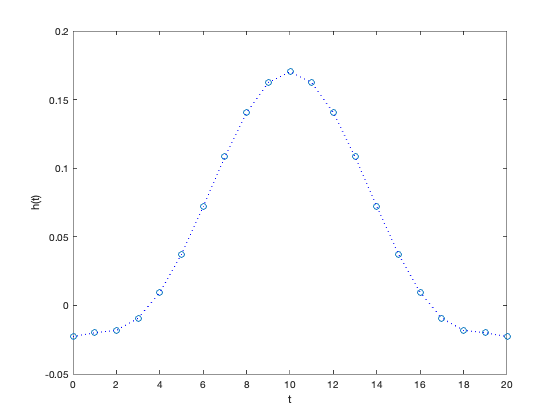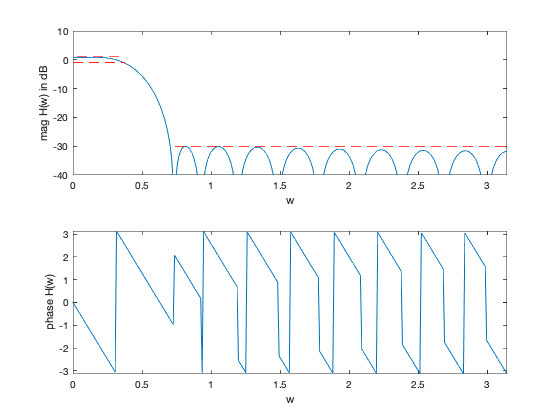```% "Filter design" lecture notes (EE364) by S. Boyd
% (figures are generated)
%
% Designs a linear phase FIR lowpass filter such that it:
% - minimizes the transition band width (i.e. minimize w_stop)
% - has a constraint on the maximum passband ripple
% - has a constraint on the maximum stopband attenuation
%
% This is a quasiconvex problem and is solved using a bisection.
%
%   minimize   w_stop
%       s.t.   1/delta <= H(w) <= delta     for w in the passband
%              |H(w)| <= atten_level        for w in the stopband
%
% where H is the frequency response function and variable is
% the filter impulse response h (and its order/length).
% Data is delta (max passband ripple) and atten_level (max stopband
% attenuation level).
%
% Written for CVX by Almir Mutapcic 02/02/06

%********************************************************************
% user's filter specifications
%********************************************************************
% starting point for the stopband (needs to be feasible)
wstop = 0.24*pi;        % stopband start freq (in radians)
TOL = 1e-3;             % precision to which we should run bisection

n = 10;                 % filter order (2n+1 is the full order)
wpass = 0.12*pi;        % passband cutoff freq (in radians)
delta = 1;              % max (+/-) passband ripple in dB
atten_level = -30;      % stopband attenuation level in dB

%********************************************************************
% create optimization parameters
%********************************************************************
m = 30*n; % freq samples (rule-of-thumb)
w = linspace(0,pi,m);

%*********************************************************************
% use bisection algorithm to solve the problem
%*********************************************************************

wstop_bot = wpass;
wstop_top  = wstop;

while( wstop_top - wstop_bot > TOL)
% try to find a feasible design for given specs
wstop_cur = (wstop_top + wstop_bot)/2;

% create optimization matrices (matrix of cosines)
A = [ones(m,1) 2*cos(kron(w',[1:n]))];

% passband 0 <= w <= w_pass
ind = find((0 <= w) & (w <= wpass));    % passband
Ap  = A(ind,:);

% transition band is not constrained (w_pass <= w <= w_stop)

% stopband (w_stop <= w) (this is the changing constraint)
ind = find((wstop_cur <= w) & (w <= pi));   % stopband
As  = A(ind,:);

% formulate and solve the feasibility linear-phase lp filter design
cvx_begin quiet
variable h_cur(n+1,1);
% feasibility problem
% passband bounds
Ap*h_cur <= 10^(delta/20);
Ap*h_cur >= 10^(-delta/20);
% stopband bounds
abs( As*h_cur ) <= 10^(atten_level/20);
cvx_end

% bisection
if strfind(cvx_status,'Solved') % feasible
fprintf(1,'Problem is feasible for stopband freq = %3.4f rads\n',wstop_cur);
wstop_top = wstop_cur;
% construct the full impulse response
h = [flipud(h_cur(2:end)); h_cur];
else % not feasible
fprintf(1,'Problem is not feasible for stopband freq = %3.4f rads\n',wstop_cur);
wstop_bot = wstop_cur;
end
end

wstop = wstop_top;
fprintf(1,['\nOptimum stopband frequency for given specs is %3.4f*pi rads\n' ...
'and the minimum transition width is %3.4f*pi radians.\n'],...
wstop/pi, (wstop-wpass)/pi);

%********************************************************************
% plots
%********************************************************************
figure(1)
% FIR impulse response
plot([0:2*n],h','o',[0:2*n],h','b:')
xlabel('t'), ylabel('h(t)')

figure(2)
% frequency response
H = exp(-j*kron(w',[0:2*n]))*h;
% magnitude
subplot(2,1,1)
plot(w,20*log10(abs(H)),...
[wstop pi],[atten_level atten_level],'r--',...
[0 wpass],[delta delta],'r--',...
[0 wpass],[-delta -delta],'r--');
axis([0,pi,-40,10])
xlabel('w'), ylabel('mag H(w) in dB')
% phase
subplot(2,1,2)
plot(w,angle(H))
axis([0,pi,-pi,pi])
xlabel('w'), ylabel('phase H(w)')
```
```Problem is not feasible for stopband freq = 0.5655 rads
Problem is not feasible for stopband freq = 0.6597 rads
Problem is feasible for stopband freq = 0.7069 rads
Problem is not feasible for stopband freq = 0.6833 rads
Problem is feasible for stopband freq = 0.6951 rads
Problem is not feasible for stopband freq = 0.6892 rads
Problem is not feasible for stopband freq = 0.6921 rads
Problem is feasible for stopband freq = 0.6936 rads
Problem is not feasible for stopband freq = 0.6929 rads

Optimum stopband frequency for given specs is 0.2208*pi rads
and the minimum transition width is 0.1008*pi radians.
```